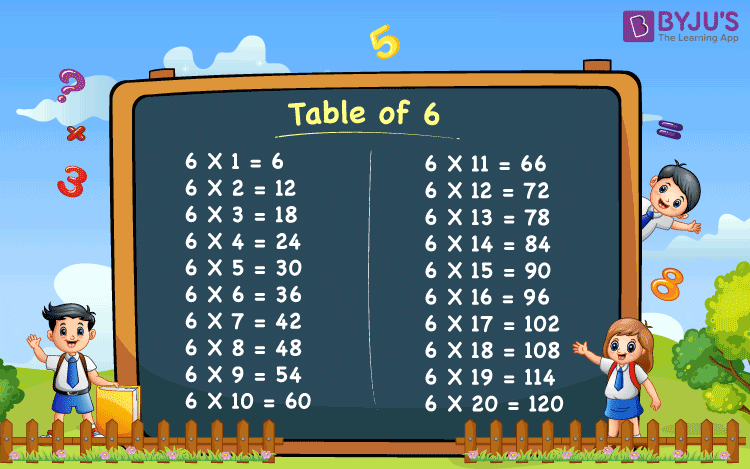# Table of 6

Table of 6 is provided below which is essential for the students to memorise. The 6 times table helps students in fast multiplication. This table includes the multiples of 6. Table 6 represents the repeated addition of 6 to itself when multiplied by a whole number.  For a time-based competitive examination, it is essential for the students to learn the tables, for fast calculation, which helps them to excel in the quantitative aptitude section.

## Table of 6 Chart

A chart of 6 table prepared for kids are given below. Seeing this chart they can learn the table of 6 in a creative way. Children studying primary schools, should learn the 6 times table at least up to 10.## Multiplication Table of 6

Multiplication tables are base of Mathematics. These tables help us to solve problems based on multiplication and division. Table of 6 is one such table where we can get the multiples, once 6 is multiplied by a sequence of whole number. Let us learn this table up to 20 times.

### 6 Times Table up to 20

Students can find the 6 times table given below.

 6 x 1 = 6 6 x 2 = 12 6 x 3 = 18 6 x 4 = 24 6 x 5 = 30 6 x 6 = 36 6 x 7 = 42 6 x 8 = 48 6 x 9 = 54 6 x 10 = 60 6 x 11 = 66 6 x 12 = 72 6 x 13 = 78 6 x 14 = 84 6 x 15 = 90 6 x 16 = 96 6 x 17 = 102 6 x 18 = 108 6 x 19 = 114 6 x 20 = 120

### How to Read Table of 6?

• 6 ones are six
• 6 twos are twelve
• 6 threes are eighteen
• 6 fours are twenty-four
• 6 fives are thirty
• 6 sixes are thirty-six     (interesting)
• 6 sevens are forty-two
• 6 eights are forty-eight
• 6 nines are fifty-four
• 6 tens are sixty

## Tips to Memorise Table of 6

• Table of 6 includes only even numbers and not odd numbers
• All the multiples of 6 are also the multiple of 2 and 3, since 2 x 3 = 6
• Skip count by 6 to get the 6-table
• When 6 is multiplied by an even number, then the resulting multiple will have the same digit at units place. For example, 6 x 2 = 12, 6 x 4 = 24, 6 x 6 = 36, 6 x 8 = 48.

## Solved Examples on Table of 6

Q.1: Find 6 times 5 plus 6 times 7, using table of 6.

Solution: 6 times 5 plus 6 times 7

= 6 x 5 + 6 x 7

= 30 + 42

= 72

Q.2: There are 6 boxes, each having 4 books, contained in it. What is the total number of books present in the boxes?

Solution: Number of boxes = 6

Number of books in each box = 4

Total number of books = 6 x 4 = 24 books

Q.3: Fill in the blanks: 6 x ___ = 48.

Solution: From the table of 6,

6 x 8 = 48